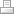# 4th Level Mathematics Online Learning Portal...

The following student portal is to provide a platform for all students to improve their performance in CfE 4th Level Mathematics...and beyond! Make sure you have a pen and paper handy, you're going to need it! You can use this section for consolidation of classwork, extension or even catching up on work missed. Ask your teacher for the password and click on the buttons below for access to the secure area...

Schools interested in a license should contact sales@cdmasterworks.co.uk with their enquiries.

Skills developed in this portal;

MTH 403c

1. The Order of Operations

MTH 406a

1. Evaluating powers/Simplifying surds
2. Calculating roots/rationalising the denominator

MTH 406b

1. Changing big numbers into scientific notation
2. Changing small numbers into scientific notation
3. Writing scientific notation as big numbers
4. Writing scientific notation as small numbers

MTH 407b

2. Multiplying fractions
3. Dividing fractions

MTH 411b

1. Calculating the surface area of a cube, cuboid and cylinder

MTH 411c

1. Calculating the volume of a cyclinder
2. Calculating the volume of a cone
3. Calculating the volume of a sphere

MTH 413a

1. Linear patterns/Calculating the nth term

MTH 413b

MTH 413c

1. Writing the equation of a straight line

MTH 413d

1. Drawing a straight line from a table of values

MTH 414a

1. Single brackets
2. Double brackets
3. Triple brackets

MTH 414b

1. Common Factor
2. Difference of 2 sqaures
3. Trinomials

MTH 415a

1. Solving equations
2. Solving inequalities

MTH 416a

1. Pythagoras' Theorem
2. Right angled Trigonometry

MTH 416b

1. Circumference of a circle
2. Area of a circle

MTH 417a

1. Interior exterior angles of polygons/Angles in a circle

MTH 417b

1. Similar Shapes - length
2. Similar Shapes - area
3. Similar Shapes - volume

MTH 418a/b

2. Plotting coordinates

MTH 419a

1. Rotational symmetry of order 2 and 4

MTH 420b

1. Calculating the range/Harder mean problems
2. Calculating the mean
3. Calculating the median
4. Calculating the mode

MTH 421a

1. Drawing Bar Graphs, Stem & Leaf Diagrams and Pie Charts

# 4th Level Pupil Recording Grid...

Feel free to download our pupil recording grid to help you log your progress in all video tutorials, worksheets and assessments. Simply enter your score as a percentage, e.g. if you scored 63% then enter 63. Also record the number of minutes it took you to complete a task, e.g. if 15 minutes were taken enter 15 in the relevant space. The grid automatically calculates many useful features which will help you with your revsion.

Only got 20 minutes spare to study? That's great! Pick a skill and start your stopwatch. Studies have shown that short, regular, productive practice is the key to success! Download your pupil record below and start your Rigour Maths "HOW MANY MINUTES???" challenge today...

CfE 4th Level Pupil Record.xlsx
Microsoft Excel sheet [99.7 KB]
S3 29 01 2019.pdf
Member LoginForgot? Show
Stay Logged In
My ProfileJavascript Required

## Calling all Parents & Students!Print | Sitemap